### PAPER:

The use of statistical tests to calibrate the Black-Scholes asset dynamics model applied to pricing options with uncertain volatility

## 1.  Abstract

A new method to solve the calibration problem for the Black-Scholes asset price dynamics model is proposed. The data used in the calibration problem are the observations of the asset price on a finite set of equispaced (known) discrete time values. Statistical tests are used to obtain estimates with statistical significance of the two parameters of the Black-Scholes model, that is of the volatility and of the drift. The consequences of these estimates on the option pricing problem are investigated. In particular the pricing problem for options with uncertain volatility in the Black-Scholes framework is revisited and a statistical significance is associated to the price intervals determined using the Black-Scholes-Barenblatt (BSB) equations. Numerical experiments with synthetic and real data are presented. The real data considered are the daily closing values of the S&P500 index and of the associated European call and put option prices in the year 2005. The method proposed to calibrate the Black-Scholes dynamics model can be extended to other models defined as stochastic dynamical systems used in science and engineering. A detailed exposition of the material summarized in this website can be found in . A general reference to the work of the authors and of their coauthors in mathematical finance is the website: http://www.econ.univpm.it/recchioni/finance.

Acknowledgments:

· The research reported in this paper is partially supported by MUR - Ministero Università e Ricerca (Roma, Italy), 40%, 2007, under grant: “The impact of population ageing on financial markets, intermediaries and financial stability”. The support and sponsorship of MUR are gratefully acknowledged.

· The numerical experience reported in this paper has been obtained using the computing grid of ENEA (Roma, Italy). The support and sponsorship of ENEA are gratefully acknowledged.

## 2.  Introduction

The Black-Scholes formulae  used to price European call and put options are based on an asset price dynamics model. This model is a stochastic dynamical system written as a stochastic differential equation. The solution of this model is a stochastic process called geometric Brownian motion. The model contains two real parameters: the volatility and the drift. The Black-Scholes formulae depend on the volatility parameter. The knowledge of the values of the volatility and of the drift is necessary to use the model to forecast asset and options prices. Therefore in practical circumstances the values of these parameters must be determined before using the Black-Scholes dynamics model and the option pricing formulae derived from it. That is, starting from the available data, the problem of finding estimates of the parameters appearing in the asset price dynamics model must be considered. This problem is called calibration problem and is an inverse problem for a stochastic dynamical system defined by a stochastic differential equation. The estimated parameter values, obtained solving the calibration problem, are used to forecast the asset price at future times and to evaluate the option pricing formulae instead than the “true” (unknown) parameter values. We can conclude that the “accuracy” and the reliability of the estimated parameter values concur to determine the accuracy and the reliability of the asset prices forecasted and of the option prices computed and/or forecasted.

Note that in recent years the validity of the asset price dynamics model proposed by Black and Scholes in 1973  has been disputed in the mathematical finance literature and that several more refined models, such as, for example, , have been introduced to describe the asset price dynamics. Nevertheless the Black-Scholes asset dynamics model and particularly the option pricing formulae derived from it remain widely used in the common practice of the financial markets. We will see in Section 3 and 4 that for the Black-Scholes model the solution of the calibration problem using statistical tests and the investigation of its consequences in the option pricing problem can be done easily using elementary mathematics. For this reason the Black-Scholes asset dynamics model is the natural choice to begin our study on the solution of calibration problems for stochastic dynamical systems using statistical tests.

The data used in the calibration problem are the observations of the asset price on a finite set of equispaced (known) discrete time values. We show how to use elementary statistical tests (that is the Student's T and the c2 tests) in order to provide values of the drift and of the volatility parameters of the Black-Scholes model with a statistical significance associated. For the convenience of the reader we recall that in a statistical test the statistical significance a, 0 < a < 1, is the maximum probability of rejecting the (null) hypothesis of the test when the hypothesis is true. Let us describe more in detail the work summarized in this website and presented in .

As already mentioned the solution of the Black-Scholes asset price dynamics model is a stochastic process called geometric Brownian motion depending from two parameters, the drift and the volatility. From this fact it follows that the asset price at any given time is modeled as a random variable with log-normal distribution and therefore the log-return of the asset price is normally distributed. Moreover it is easy to see that the asset price log-return increments associated to observations of the asset price made on a (finite) set of equispaced time values are a sequence of values sampled from a set of independent identically distributed Gaussian random variables. The mean and the variance of these Gaussian random variables can be written with elementary formulae as a function of the drift and of the volatility parameters of the Black-Scholes asset price dynamics model and of the time interval between the observations. That is, starting from a sample of observations of the log-return increments on a finite set of equispaced (known) time values it is possible using elementary statistical tests (i.e.: Student's T and c2 tests) to estimate, with a given (statistical) significance, the mean and the variance of the Gaussian random variables associated to the log-return increments. In fact the Student's T test (, ) is the test used to establish with statistical significance whether the mean of a normally distributed population made of independent samples has a value or belongs to an interval specified in a “null” hypothesis. Similarly the c2 test (, ) is the test used to establish with statistical significance whether the variance of a normally distributed population made of independent samples has a value or belongs to an interval specified in a “null” hypothesis. Moreover from the knowledge of the parameters of the Gaussian random variables associated to the log-return increments derived from the statistical tests it is easy to recover the corresponding knowledge of the parameters of the Black-Scholes model and to associate to it a statistical significance.

The use of statistical tests in the solution of the calibration problem for stochastic dynamical systems, such as the Black-Scholes model, is an interesting way of approaching these inverse problems that can be used elsewhere in mathematical finance and in many application contexts different from mathematical finance. The significance levels obtained for the parameter values (intervals) of the Black-Scholes model are relevant in many practical circumstances. In Section 4 we consider one of these circumstances: the pricing problem for options with uncertain volatility. The pricing problem for options with uncertain volatility has been considered by several authors in the scientific literature. For simplicity we refer to  and  where, in the Black-Scholes framework, assuming that the (uncertain) volatility belongs to a known interval, the corresponding price intervals for the (European vanilla) option prices are determined using the Black-Scholes-Barenblatt (BSB) equations. Thank to our methodology statistical significance levels can be attributed to the option price intervals determined using the BSB equations.

Finally the results obtained in the calibration problem for the Black-Scholes model are applied to the study of synthetic and real data. The synthetic data considered are generated integrating numerically the stochastic differential equation that defines the asset price dynamics in the Black-Scholes model for several choices of the parameter values. The real data studied are the time series of the daily closing values of the S&P500 index and of the associated European vanilla option prices in the year 2005. The numerical results obtained are computationally simple and statistically convincing.

In mathematical finance the problem of estimating the volatility of the asset price starting from a time series of observed data (asset and/or option prices) has received great attention. The most common methods used in the literature are: the implied and the historical (or realized) volatility methods. The approach proposed here to solve this problem is different from these methods. Unlike in the implied volatility method we do not consider the volatility implied by the option prices observed in the market. That is we don't estimate the volatility parameter of the asset price using as data the prices of a variety of options on the asset with different strikes and expiration dates. On the other hand, as in the historical volatility method, our method estimates the volatility starting from the asset prices observed on a finite set of discrete time values. Our method improves with respect to the historical volatility method since associates a significance level to the volatility estimate obtained. In some sense going from the historical volatility method to the method proposed here corresponds to going from the use as volatility estimate of the “sample volatility” to the use of a statistical test (i.e. the c2 test) tailored on the random variable whose parameters must be estimated. That is, roughly speaking, it corresponds to going from a method of descriptive statistics to a method of mathematical statistics.

Let us mention some valuable features of the work presented here. First of all the idea proposed is very simple. Although the idea is introduced in the context of the Black-Scholes model, it can be applied to other stochastic dynamical systems used in mathematical finance to describe asset price dynamics. For example we have in mind the Heston model (see ,  ), or some of its variations such as the models introduced in , , ,  to study some specific problems. In , , , , ,  the calibration problem for the models considered is studied using the “implied volatility method” or the maximum likelihood method. In those papers there are not statistical tests involved in the solution of the calibration problems and to the estimated parameter values no statistical significance is associated. Note that in the study of the calibration problem for these models, or, more in general, for a generic stochastic dynamical system no elementary statistical tests (such as Student's T and c2 tests) can be used. In order to extend the method suggested here to the study of these more general dynamical systems new ad hoc statistical tests must be developed. Most likely in order to carry out these new statistical tests on a data sample a substantial use of numerical methods will be necessary.

A general reference to the work of the authors and of their coauthors in mathematical finance is the website: http://www.econ.univpm.it/recchioni/finance.

## 3.  The calibration problem for the Black-Scholes model

Let St > 0 denote the asset price at time t ³ 0. The Black-Scholes model   assumes that St, t > 0, is a stochastic process whose dynamics is governed by the following stochastic differential equation: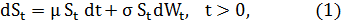with the initial condition: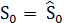, (2)

where m, s are real parameters, m is the drift, s > 0 is the volatility, Wt, t > 0, is the standard Wiener process, W0 = 0, dWt, t > 0, is its stochastic differential and the initial condition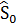> 0 is a given random variable. For simplicity we assume thatis a random variable concentrated in a point with probability one, and, with abuse of notation, we denote this point with> 0. The parameters m, s are the unknowns of the calibration problem.

The stochastic differential equation (1) defines the so called geometric Brownian motion. Let ln(·) denote the logarithm of ·, for t ³ 0 the quantity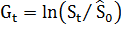is called log-return at time t of the asset whose price is St. It is easy to see that the Black-Scholes asset price log-return increments associated to a discrete set of equispaced time values are a sequence of independent identically distributed Gaussian random variables. In particular let Dt > 0 be a time increment and let ti = i Dt, i = 0,1,¼,n, be a discrete set of equispaced time values that later will be chosen as the set of the observation times. We define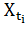, the asset price log-return increment when t goes from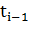to ti as follows: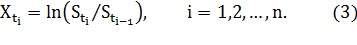It is easy to see (see  for more details)  that the random variables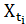,, i = 1,2,¼,n, are independent identically distributed Gaussian random variables with mean M and variance V2 where: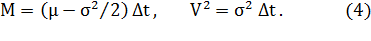We consider the following calibration problem: given Dt > 0, a statistical significance level a, 0 < a < 1, and the asset price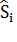observed at time t = ti = i Dt, i = 0,1,¼,n, determine two intervals where the parameters m and s of the model (1) respectively belong with the given significance level a.

The observations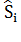of the asset price at time t = ti, i = 0,1,¼,n, are non negative real numbers that we assume not affected by errors. These n+1 observations of the asset price and the corresponding time values are the data used in the calibration problem. The observed log-return increments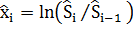, i = 1,2,¼,n, are a sample of n observations taken respectively from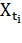, i = 1,2,¼,n, that is taken from a set of independent identically distributed Gaussian random variables. Using this data sample, through the Student's T test and the c2 test respectively (see , , ), we determine two intervals where the mean M and the variance V2 of the Gaussian random variables mentioned above belong with the given significance level a. From the knowledge of the intervals determined for M and V2, the corresponding intervals for m and s can be recovered inverting the relations (4).

In particular given a significance level a, 0 < a < 1, we can perform statistical tests on the variance V2 and on the mean M of the random variables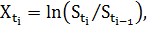i = 1,2,¼,n, starting from the data sample, i = 1,2, ¼,n, using the c2 test and the Student's T test respectively. This implies that given a, 0 < a < 1, and given four real numbers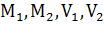such that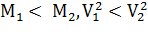we can accept or reject, with significance level a, the hypotheses: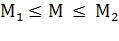,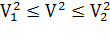,    (5)

and, consequently, using the relations (4) , we can accept or reject, with significance level a, the hypotheses: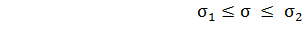,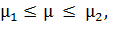(6)

where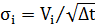,   and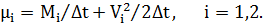(7)

Relations (7) are obtained simply translating to s and m the results on V2 and M obtained with the statistical tests. For more details see .

To estimate V2 and M from the log-return increments we must choose the hypotheses to test. This can be done in many ways. The results on synthetic and real data shown in Section 5 are obtained using a set of heuristic rules to choose the hypotheses to be tested. These rules and the way of using them is defined in the Procedures 1 and 2 described in detail in .

## 4.  Option prices with uncertain volatility and statistical significance

Let us consider the Black-Scholes asset price dynamics model (1) and the problem of pricing options with uncertain volatility in the Black-Scholes model with a given statistical significance a, 0 < a < 1. We assume that the volatility value s is not known exactly, but that it is known that the volatility lies in a specified range, let us say s1 £ s £ s2, where s1, s2 are constants and 0 < s1 < s2, with a given significance level a. In fact, using the c2 test on the data of the calibration problem posed in Section 3, we can decide whether to accept or to reject the statement:

 s1 £ s £ s2,      with significance level  a.

(8)

We limit our attention to European vanilla call and put options.

The question that we want to answer is: given a significance level a, 0 < a < 1, assuming that the hypothesis  s1 £ s £ s2 is accepted with significance level a, determine the corresponding range where the value of an European vanilla option lies (with significance level a).  The answer to this question follows from the work of Avellaneda, Levy and Parás  and of Lyons . These authors propose a way to price options in the Black-Scholes model when the volatility s is not known exactly, but it is simply known that:

 s1 £ s £ s2.

(9)

In  and   significance levels are not considered.  We remark that the bounds s1 and s2 in the two equations (8) and (9) are determined in different ways. In (8) they are parameters that can be chosen together with a significance level in such a way that on the data sample considered the c2 test will accept the hypothesis  s1 £ s £ s2 with significance level a, or are parameters determined starting from the data sample through an iterative procedure such as Procedure 1 described in . In (9) these bounds are assigned using common sense assumptions or they are determined either looking at extreme values of the volatility implied by the observed option prices or looking at the low-high peaks of the historical volatility.

Let t be the time variable, S be the asset price, τ> 0 be the expiration date of the option to be priced. In  and   it is shown that, in the Black-Scholes framework, when the volatility satisfies (9), there exists an interval [Ʋ1, Ʋ2] depending on S and t, such that the price Ʋ = Ʋ(S,t), S > 0, 0 < t £ τ, of the option lies in this interval, that is (9) implies that:

 Ʋ1(S,t) £ Ʋ(S,t) £ Ʋ2(S,t),     S > 0,     0 < t £ τ.        (10)

The worst-case option value Ʋ1(S,t),  S > 0,  0 < t £ τ, and the best-case option value Ʋ2(S,t), S > 0, 0 < t £ τ, satisfy two nonlinear partial differential equations known as Black-Scholes-Barenblatt (BSB) equations. The BSB equations reduce to the usual Black-Scholes equation when there is no uncertainty about the volatility value (i.e. in the case s1 = s2). For a general pay-off function the BSB equations do not have a closed-form solution and must be solved numerically. However due to the properties of the BSB equations when a call or a put option is considered, the BSB equations reduce to the Black-Scholes equation. See  and   for more details. We briefly recall that the Black-Scholes equation is linear and, when simple final conditions, as the ones corresponding to call and put options, are imposed, can be solved explicitly leading to the Black-Scholes formulae (see ).

Now we can answer the question posed at the beginning of this Section. First of all let us assume that a “true” value of the volatility s exists even if it is unknown. From the fact that in the Black-Scholes model the price Ʋ of an option is a monotonically increasing function of the volatility s we can conclude that when (8) holds we have:

 “Ʋ1(S,t) £ Ʋ(S,t) £ Ʋ2(S,t),     S > 0,  0 < t <  τ   with significance level  a”,

(11)

where Ʋ1(S,t),  Ʋ2(S,t), S > 0,  0 < t < τ, are the solutions of the appropriate BSB equations.

The meaning of the sentence (11) can be restated as follows: if we assume that a “true” value of the volatility s exists and that based on the analysis of a data sample we accept the hypothesis that this true value lies in the range [s1, s2] with significance level a, i.e. s1 £ s £ s2 with probability 1-a, it follows that the corresponding “true” value of the option price Ʋ lies in the range [Ʋ1, Ʋ2] with probability 1-a, i.e. “Ʋ1(S,t) £ Ʋ(S,t) £ Ʋ2(S,t), S > 0,  0 < t < τ, with significance level a”, where Ʋ1,  Ʋ2, are the solutions of the appropriate BSB equations.

## 5.  Numerical experiments

Let us discuss some numerical experiments. The first numerical experiment presented consists in solving the calibration problem discussed in Section 3 using synthetic data. In this experiment the analysis of a time series of daily data of the asset prices during a period of ten years is considered. We assume that a year is made of 253 trading days. The time series studied is made of 253·10+1=2531 daily asset price data, that is it is made of the asset priceobserved at time t = ti = i Dt, i = 0,1,¼, 2530, Dt = 1/253. These synthetic data have been obtained computing one trajectory of the stochastic differential equation (1) for several choices of the parameter values and looking at the computed trajectory at time t = ti = i Dt, i = 0,1,¼, 2530. We have chosen as initial condition at time t = t0 = 0, S0 =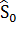= 1200. We choose m =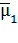= 0.01 and s =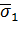= 0.1 in the first five years of data (i.e. when t = ti, i = 0,1,¼,1264), m =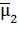= 0.06 and s =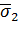= 0.4 in the sixth and seventh year of data (i.e. when t = ti, i = 1265,1266,¼,1770) and m =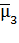= 0.03 and s =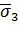= 0.2 in the last three years of data (i.e. when t = ti, i = 1771,1772¼,2530). The synthetic data are generated using as initial value of the sixth year of data the last datum of the fifth year of data. A similar statement holds for the initial value of the last three years of data. The daily log-return increments of the synthetic asset prices generated in this way are shown in Figure 1. The fact that the data are generated using three different choices of parameter values can be seen simply looking at Figure 1.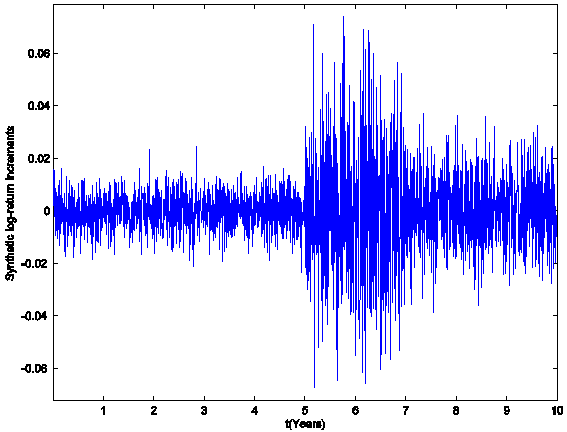Figure 1: The daily log-return increments (synthetic data).

We consider the following calibration problem: given Dt = 1/253, a = 0.1, and given the asset price observationat time t = ti = i Dt, i = 0,1,¼,2530, determine the intervals where the parameters m and s of model (1) belong with significance level a = 0.1.

We solve this calibration problem with Procedures 1 and 2 described in  using the data associated to a time window made of 253 consecutive observation times, that is the observations corresponding to 253 consecutive trading days (one year), and we move this window across the ten years of data discarding the datum corresponding to the first observation time of the window and inserting the datum corresponding to the next observation time after the window. The calibration problem is solved for each choice of the data time window applying Procedures 1 and 2 described in , that is it is solved 2530-253+1=2278 times finding for each calibration problem solved two intervals where the volatility and the drift parameters respectively belong with significance level a = 0.1. The reconstructions of the parameters obtained moving the window along the data as specified above are shown in Figure 2, Animation 1 and Animation 2. Figure 2, Animation 1 and Animation 2 show the “true” values of the parameters and the intervals determined using the statistical tests on the data windows via Procedures 1 and 2 of . In particular Animation 1 (Animation 2) is a digital movie showing the parameter s2 (m)  reconstructed from the synthetic data. In Figure 2, Animation 1 and Animation 2 the abscissa corresponds to the data window used to reconstruct the model parameters. The data windows are numbered in ascending order beginning with one according to the order in time of the first day of the window considered. In particular Figure 2, Animation 1 and Animation 2 show that the intervals containing the parameters m and s and the time when the parameter values change are reconstructed satisfactorily.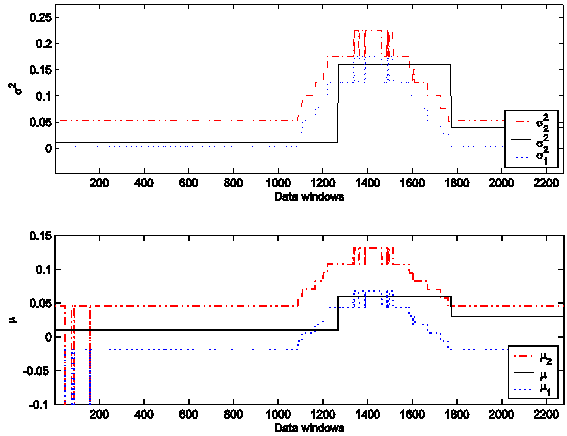Figure 2: The parameters s2 and m reconstructed from the synthetic data.

The second numerical experiment uses real data. The real data studied are the 2005 daily data of the U.S. S&P500 index (see Figure 3) and of the prices of European vanilla call and put options on this index.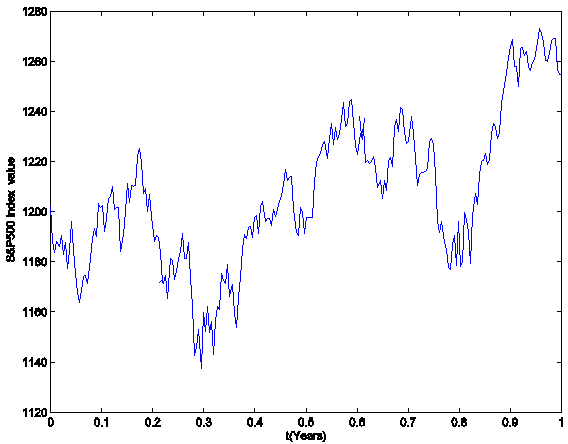Figure 3: The S&P500 index (year 2005).

We remind that the U.S. S&P500 index is one of the leading indices of the New York Stock Exchange. More specifically we consider the daily closing values of the S&P500 index and of the bid prices of the vanilla European call and put options on the S&P500 index during the period of about 12 months going from January 3, 2005 to December 30, 2005. In this period we have 253 trading days and more than 153.000 option prices. We limit our study to the call and the put prices corresponding to options that have a positive volume (i.e. a positive number of contracts) traded the day corresponding to the price considered and a positive bid price. These are 46.823 options prices. Since there are 253 trading days in the year 2005 we choose as time unit a “year” made of 253 trading days. We choose as time t = t0 = 0 the day January 3, 2005. We have 253 daily S&P500 index valuesobserved at time t = ti = i Dt, i = 0,1,¼,252, with Dt = 1/253 year. The S&P500 index and the corresponding (daily) log-return increments in the year 2005 are shown in Figure 3 and Figure 4 respectively.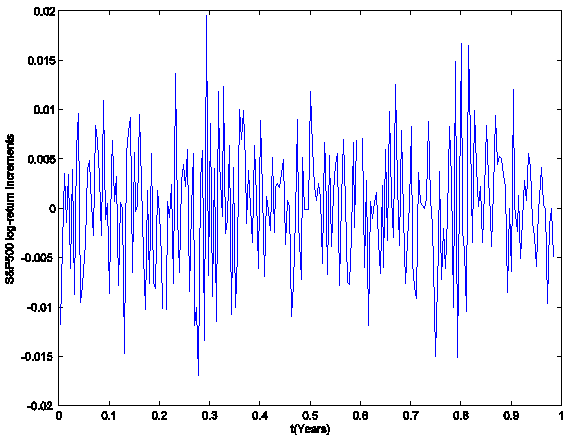Figure 4: The S&P500 daily log-return increments (year 2005).

We interpret this set of data using the Black-Scholes model as suggested in Section 3. We begin studying the variance and the drift of the Black-Scholes model used to explain S&P500 index during the year 2005. The S&P500 daily log-return increments, i = 1,2,¼,252, are analyzed using the Black-Scholes model, that is they are considered as a sample (see Figure 4) of 252 observations taken from a set of independent identically distributed Gaussian random variables where the mean M and the variance V2 are defined in (4). First we estimate V2 (and therefore s2) using the c2 test and subsequently we estimate M (and therefore m) using the Student's T test using Procedures 1 and 2 described in .

Given the data sample made of the S&P500 daily log-return increments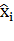, i = 1,2,¼,252, the significance level a = 0.1, and appropriate initial values to start the Procedures 1 and 2 described in  we find that the hypotheses:

 2.5297 ·10-3 = s12 £ s2 £ s22 = 2.7232 ·10-2 ,       (12)

 -1.1087 ·10-2 = m1 £ m £ m2 = 2.5968 ·10-2,

(13)

are accepted with significance level a = 0.1.

Let us do a kind of stability analysis of the intervals (12), (13) determined with the statistical tests. To do this we fix a = 0.1 and we apply Procedures 1 and 2 described in  to determine the intervals where s2 and m belong with significance level a starting from a window of 70 consecutive observations corresponding to 70 consecutive observation times (i.e. 70 consecutive trading days). As done before we move the data window through the data time series discarding the datum corresponding to the first observation time of the window and inserting the datum corresponding to the next observation time after the window. Proceeding in this way we have 252-70+1 data windows in the data time series considered and for each one of these data windows we solve the corresponding calibration problem. We find 252-70+1 couples of intervals where the volatility and the drift parameter belong with significance level a = 0.1. Figure 5 shows that, changing the data window, the intervals determined through Procedures 1 and 2 remain stable. In Figure 5 the abscissa represents the data windows used to reconstruct the model parameters numbered in ascending order beginning with one according to the order in time of the first day of the window considered. In correspondence to each data window the intervals determined by Procedures 1 and 2 of  are shown.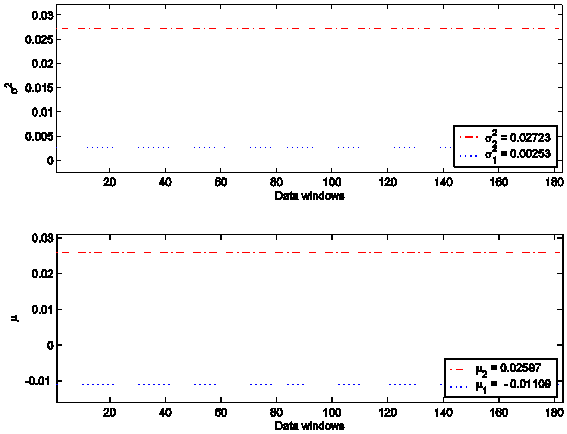Figure 5: The parameters s2 and m reconstructed from the S&P500 data (year 2005).

Finally we consider the 46.823 S&P500 European call and put option prices of the year 2005 already mentioned and we try to interpret them using the method developed in Section 4. From the estimates (6) established with significance level a = 0.1, we are able to determine the corresponding option price intervals using the BSB equations. That is we can determine the worst-case option values Ʋ1(S,t), S > 0, 0 < t £ τ, and the best-case option values Ʋ2(S,t), S > 0, 0 < t £ τ. We compute the percentage of the prices of the European call (% call) and put (% put) options on the S&P500 observed in the year 2005 that verify (11) when we assume the first relation in (6). The results obtained are shown in Tables 1-3. In these tables Ncall and Nput denote respectively the number of the call prices and of the put prices corresponding to options whose characteristics are described in the caption of the table. The quantities Icall and Iput denote respectively the average relative amplitude of the call price intervals and of the put price intervals determined using the BSB equations, and Pcall, Pput denote respectively the average bid price of the call and of the put prices. In parentheses in the % call and % put columns it is written the average number of contracts on the options considered traded.

 January-April 2005 % call % put Ncall Nput Icall Iput Pcall Pput 73.8 % (172.22) 72.3 % (313.01) 1571 1822 0.23 0.34 94.01 76.82 May-August 2005 % call % put Ncall Nput Icall Iput Pcall Pput 74.6 % (335.25) 62.0 % (202.34) 2005 1745 0.24 0.35 92.03 76.73 September-December 2005 % call % put Ncall Nput Icall Iput Pcall Pput 65.5 % (401.78) 59.7 % (557.22) 2174 2300 0.23 0.35 97.94 76.14

Table 1: S&P500 option prices: in the money options (year 2005). These results are obtained using estimates (12), (13).

 January-April 2005 % call % put Ncall Nput Icall Iput Pcall Pput 51.1 % (1358.18) 67.7 % (1869.60) 852 902 0.27 0.28 28.29 24.76 May-August 2005 % call % put Ncall Nput Icall Iput Pcall Pput 56.5 % (1899.99) 66.2 % (1989.07) 1115 1154 0.28 0.28 27.40 22.52 September-December 2005 % call % put Ncall Nput Icall Iput Pcall Pput 41.5 % (2377.19) 59.4 % (2652.84) 1188 1208 0.27 0.28 30.29 23.45

Table 2: S&P500 option prices: at the money options (year 2005). These results are obtained using estimates (14), (15).

 January-April 2005 % call % put Ncall Nput Icall Iput Pcall Pput 20.1% (691.52) 2.41 % (1061.76) 3412 4892 0.26 0.57 11.13 9.05 May-August 2005 % call % put Ncall Nput Icall Iput Pcall Pput 13.4% (929.68) 3.55 % (1337.39) 3644 6316 0.23 0.58 9.89 8.33 September-December 2005 % call % put Ncall Nput Icall Iput Pcall Pput 12.6% (1598.65) 2.71 % (1908.66) 4055 6468 0.24 0.59 12.73 8.58

Table 3: S&P500 option prices: out of the money options (year 2005). These results are obtained using estimates (16), (17).

We recall that given the asset price S and the strike price K of an option, a call option (a put option) is in the money if S > K (if S < K), is out the money if S < K (if S > K) and is at the money if S = K. In the numerical experiments the condition S = K is substituted with |S-K| < e where e is a (given) positive quantity. As a consequence the conditions S > K, S < K are rewritten as S > K+e, and S < K-e, respectively. Using this criterion the 46.823 option prices considered above are divided in three subsets corresponding to prices of in the money, at the money and out of the money options. We take e equal to one per cent of the average strike price of the options considered.

Tables 1 refers to the in the money S&P500 option prices and it is obtained specifying (6) as (12), (13).

A similar analysis relative to S&P500 option prices corresponding to options out and at the money shows that the use of the intervals (12), (13) leads to huge call and put price intervals making the results obtained of dubious practical value. One way of overcoming this drawback is to refine the estimates (12), (13) changing the value of a tolerance parameter named tol used in Procedures 1 and 2 described in  until option price intervals of “acceptable average relative amplitude” (i.e. average relative amplitude of some tens of percentage points) are obtained. For more details see . Reducing by one quarter the parameter tol we find that the hypotheses:

 8.7051 ·10-3 = s12   £ s2 £  s22 = 1.4881 ·10-2 ,      (14)

 1.2646 ·10-3 = m1   £ m £  m2 = 1.0528 ·10-2,           (15)

are accepted with significance level a = 0.1. The choice of (14), (15) as intervals containing s2 and m respectively leads to average relative amplitudes of some tens of percentage points for the option price intervals when call and put options at the money are considered. Table 2 shows the results obtained on at the money option prices using (14), (15).

When S&P500 option prices relative to options out the money are considered it is necessary to reduce further the parameter tol to keep the average relative amplitude of the option price intervals to reasonable values. For example reducing further the parameter tol we find that the hypotheses:

 1.1021 ·10-2 = s12   £ s2 £  s22 = 1.2565 ·10-2 ,     (16)

 4.7385 ·10-3 = m1   £ m £  m2 = 7.0544 ·10-3,          (17)

are accepted with significance level a = 0.1. Table 3 shows the results obtained on out of the money option prices using (16), (17).

Table 1 shows that the in the money S&P500 option prices are well interpreted by the model proposed here. Percentages of the prices of the in the money S&P500 call and put options explained by the model in the 60%-80% range with average relative amplitude of the option price intervals in the 20%-40% range can be considered as a good result of possible practical value. Looking at Table 1 we see that in the case of in the money options the call prices seem to be better explained than the put prices. On the other hand if we look at the numerical results shown in Table 2 we see that the at the money S&P500 put options prices are better explained than the corresponding S&P500 call options prices. Altogether also the results relative to at the money S&P500 option prices are satisfactory. Unfortunately Table 3 shows that the out of the money S&P500 option prices and above all the S&P500 prices relative to out of the money put options are not well interpreted using our methodology. This could be related to the fact that the out of the money options have usually low prices. Tables 1-3 show that the prices of the options out of the money are the smallest ones.

## 6. References

  M.Avellaneda, A.Levy, A.Parás, “Pricing and hedging derivative securities in markets with uncertain volatilities”, Applied Mathematical Finance, 2, pp. 73-88, 1995.

  A.Azzalini, “A class of distributions which includes the normal ones”, Scandinavian Journal of Statistics, 12(2), pp. 171-178, 1985.

  F.Black, M.Scholes, “The pricing of options and corporate liabilities”, Journal of Political Economy, 81, pp. 637-659, 1973.

  F.Mariani, G.Pacelli, F.Zirilli, “Maximum likelihood estimation of the Heston stochastic volatility model using asset and option prices: an application of nonlinear filtering theory, Optimization Letters, 2, pp. 177-222, 2008.

  L. Fatone, F.Mariani, M.C.Recchioni, F.Zirilli, “Maximum likelihood estimation of the parameters of a system of stochastic differential equations that models the returns of the index of some classes of hedge funds”, Journal of Inverse and Ill Posed Problems, 15, pp. 493-526, 2007. (http://www.econ.univpm.it/recchioni/finance/w5).

  L.Fatone, F.Mariani, M.C.Recchioni, F.Zirilli, “The calibration of the Heston stochastic volatility model using filtering and maximum likelihood methods”, in Proceedings of Dynamic Systems and Applications, G.S.Ladde, N.G.Medhin, Chuang Peng, M.Sambandham Editors, Dynamic Publishers, Atlanta, Georgia, USA, 5, pp. 170-181, 2008.  (http://www.econ.univpm.it/recchioni/finance/w6).

  L.Fatone, F.Mariani, M.C.Recchioni, F.Zirilli, “An explicitly solvable multi-scale stochastic volatility model: option pricing and calibration”, Journal of Futures Markets, 29(9), pp. 862-893, 2009. (http://www.econ.univpm.it/recchioni/finance/w7).

  L.Fatone, F.Mariani, M.C.Recchioni, F.Zirilli, “Calibration of a multiscale stochastic volatility model using as data European option prices”, Mathematical Methods in Economics and Finance, 3, pp. 49-61, 2008.

  L.Fatone, F.Mariani, M.C.Recchioni, F.Zirilli, “The analysis of real data using a multiscale stochastic volatility model”, European Financial Management,doi: 10.1111/j.1468-036X.2010.00584.x, (2011). (http://www.econ.univpm.it/recchioni/finance/w9).

  L.Fatone, F.Mariani, M.C.Recchioni, F.Zirilli, “The use of statistical tests to calibrate the Black-Scholes asset dynamics model applied to pricing  options with uncertain volatility”,  Journal of  Probability and Statistics, Volume 2012, Article ID 931609, 20 pages, 2012.

  S.L.Heston, “A closed-form solution for options with stochastic volatility with applications to bond and currency options”, The Review of Financial Studies, 6(1), pp. 327-343, 1993.

  J.H.Hull, Options, Futures, and Other Derivatives, 7th Edition, Pearson Prentice Hall, London, 2008.

  T.J.Lyons, “Uncertain volatility and the risk-free synthesis of derivatives”, Applied Mathematical Finance, 2(2), pp. 117-133, 1995.

   K. Pearson, “On the criterion that a given system of deviations from the probable in the case of correlated system of variables is such that it can be reasonably supposed to have arisen from random sampling”, Philosophical Magazine, 50, pp. 157-175, 1900.

   Student [W.S.Gosset*], “The probable error of a mean”, Biometrika, 6(1), pp. 1-25, 1908.

   R.A.Johnson, G.K.Bhattacharyya, Statistics: Principles and Methods, 5th Edition, John Wiley & Sons, New York, 2006.

* From Wikipedia: “The T-statistic was introduced in 1908 by William Sealy Gosset, a chemist working for the Guinness brewery in Dublin, Ireland (“Student” was his pen name). Gosset had been hired due to Claude Guinness's innovative policy of recruiting the best graduates from Oxford and Cambridge to apply biochemistry and statistics to Guinness' industrial processes. Gosset devised the T test as a way to cheaply monitor the quality of stout. He published the test in Biometrika in 1908, but was forced to use a pen name by his employer, who regarded the fact that they were using statistics as a trade secret. In fact, Gosset's identity was unknown to fellow statisticians.”

Entry n. 2342